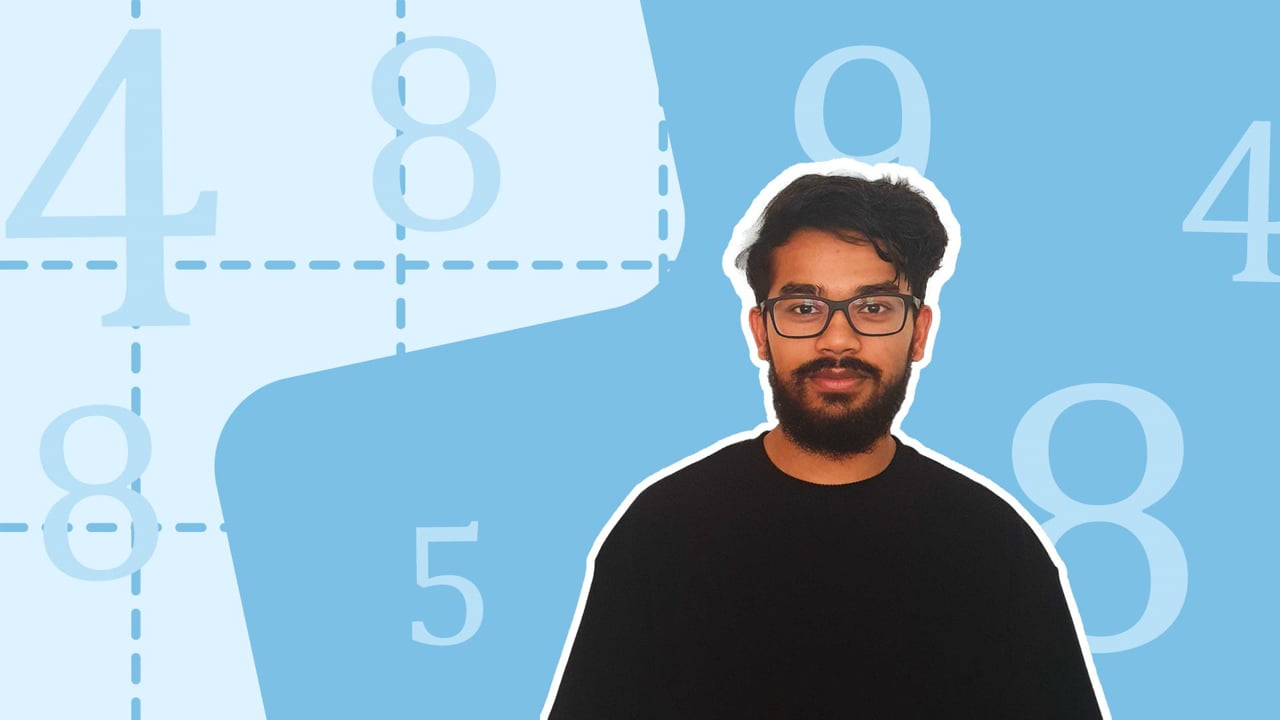Chapter overviewMaths

Number and place value

Multiplication and division

Fractions

Measurement

Geometry - properties of shapes

Geometry - position and direction

Statistics

Maths

# Dividing numbers up to 4 digits by a 1-digit number0%

Summary

# Dividing numbers up to 4 digits by a 1-digit number

## ​​​​In a nutshell

Division is the inverse of multiplication. Four digit numbers are whole numbers between $1000$​ and $9999$. They can primarily be divided by a one-digit number using two methods: chunking and short division.

## Chunking

The chunking method separates four digit numbers into multiple easy to divide chunks in order to divide a four digit number by a one digit number.

##### Example 1

Calculate: $2500 \div 5.$

​ $2500$ can be split into two smaller "chunks" of $2000$ and $500$.​

\begin{aligned} 2000\div5 &= 400 \\ 500\div5&=100 \\ \\ 400+100&=500 \\ \end{aligned}​​

$2500\div5 = \underline{500}$

Note: Use chunking for simple four digit numbers such as: $1000, 4500, 8000$.

## Short division

Short division is also known as the "bus stop" method. To perform short division:

#### PROCEDURE

 ​$1$​​ Write the number your are dividing by under the "bus stop" and the number being divided as follows: $5000\div5 \rightarrow 5\overline{\smash{)}5000}$. ​$2$​​ Starting from the left-hand side of the number being divided, find how many times the divisor goes into the first digit. ​$3$​​ Write the answer above the "bus stop" and carry over any remainders to the next digit. ​$4$​​ Repeat the procedure for the next digit.

##### Example 2

Calculate: $5740 \div 4 .$

Write $4$ outside the bus stop and $5740$ inside the bus stop. Begin by finding how many $4$s go into $5$.

$4\overline{\smash{)}5740}$

$5 \div 4= 1\space remainder \ 1$

Write the answer $1$ above $5$. Write the remainder $1$ in front of $7$. Work out how many $4$s go into $17$.

$4\overset{1\,\,\,\,\,\,\,\,\,\,\,\,}{\overline{\smash{)}5~^1\!740}}$

$17\div 4 = 4\space remainder\space 1$

​​

Write the answer $4$ above $7$. Write the remainder $1$ in front of $4$. Work out how many $4$s go into $14$.

$4\overset{\,\,1\,\,4\,\,\,\,\,\,\,\,\,\,}{\overline{\smash{)}57~^1\!40}}$

$14 \div 4 = 3 \space remainder \space 2$

Write the answer $3$ above $4$. Write the remainder $2$ before $0$. Lastly, work out how many $4$s go in $20$.

$4\overset{\,\,\,1\,\,4\,\,3\,\,\,\,\,\,\,\,}{\overline{\smash{)}574~^2\!0}}$​​

​​$20\div 4 = 5$

​​

$4\overset{\,\,\,1\,\,4\,\,3\,\,5\,}{\overline{\smash{)}574~\!0}}$

$5740 \div 5 = \underline{1435}$

​​

## Remainders

One-digit numbers do not always fit into a four digit number. The amount which is left over after division is known as a remainder.

##### Example 3

Use the chunking method to solve: $1004\div5.$

$1004$ can be split into three smaller "chunks" of $500$​​ and $500$ and $4$.

\begin{aligned} 500\div5 &= 100 \\ 500\div5&=100 \\ \\ 100+100&=200 \\ \end{aligned}

$4$​ is not divisible by $5$​.

$1004\div5 = \underline{200\space remainder\space 4}$

##### Example 4

Use short division to solve: $3964\div3$.

Write $3$ outside the bus stop and $3964$​ inside the bus stop.

$3{\overline{\smash{)}3964}}$​​

Find how many $3$​'s first fit into $3$​, $9$​ and $6$​. Write the answers, $1$​, $3$​ and $2$​ above each number respectively.

$3\overset{\,\,\,\,1\,3\,\,2\,\,\,\,}{\overline{\smash{)}3964}}$

$4$​ is not wholly divisible by $3$.

Find the largest whole division you can perform.

$3\div3=1$

​​

Write $1$ above $4$.

$3\overset{\,\,\,\,1\,3\,\,2\,\,1\,\,}{\overline{\smash{)}3964}}$​​

Find the remainder.

\begin{aligned} 3\times1 = 3 \\ 4-3=1 \end{aligned}​​

$3964\div3 = \underline{1321\space remainder\space 4}$​​

## Want to find out more? Check out these other lessons!

Multiplying 2 and 3-digit numbers by a 1-digit number

FAQs

• Question: What is a remainder?

Answer: The amount which is left over after division is known as a remainder.

• Question: What is another name for short division?

Answer: Short division is also known as the "bus stop" method.

• Question: What are the four digit numbers?

Answer: Four digit numbers are whole numbers between 1000​​ and 9999.

Theory

Exercises